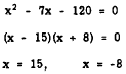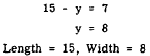Custom SearchGRAPHICAL INTERPRETATION OF ROOTS When a quadratic is set equal to y and the resulting equation is graphed, the graph will reveal the character of the roots, but it may not reveal whether the roots are rational or irrational. Consider the following equations: 1. x2 + 6x - 3 = y 2. x2 + 6x + 9 = y 3. x2 + 6x + 13 = y The graphs representing these equations are shown in figure 16-4. We recall that the roots of the equation are the values of x at those points where y is zero. Y is zero on the graph anywhere along the X axis. Thus, the roots of the equation are the positions where the graph crosses the X axis. In parabola No. 1 (fig. 16-4) we see immediately that there are two roots to the equation and that they are unequal. These roots appear to be -6.5 and 0.5. Algebraically, we find them to be the irrational numbersFor equation No. 2 (fig. 16-4), the parabola just touches the X axis at x = -3. This means that both roots of the equation are the same that is, the root is a double root. At the point where the parabola touches the X axis, the two roots of the quadratic equation have movedFigure 16-4.-Graphical interpretation of roots. together and the two points of intersection of the parabola and the X axis are coincident. The quantity -3 as a double root agrees with the algebraic solution. When the equation No. 3 (fig. 16-4) is solved algebraically, we see that the roots are -3 + 2i and -3 - 2i. Thus they are imaginary. Parabola No. 3 does not cross the X axis. When this situation occurs, imaginary roots are implied. Only equations having real roots will have graphs that cross or touch the X axis. Thus we may determine from the graph of an equation whether the roots are real or imaginary. VERBAL PROBLEMS INVOLVING QUADRATIC EQUATIONS Many practical problems give rise to quadratic equations. In such problems it often happens that one of the roots will have no meaning. We must select the root that satisfies the conditions of the problem. Consider the following example: The length of a plot of ground exceeds its width by 7 ft and the area of the plot is 120 sq ft. What are the dimensions? SOLUTION : Let x = length y = width then x - y = 7 and xy = 120 Solving (1) for y, y = x - 7 Substituting (x - 7) for y in (2) x(x - 7) = 120 ThereforeThus, length = +15 or -8. But the length obviously cannot be a negative value. Therefore, we reject -8 as a value, for x and use only the positive value, +15. Then from equation (1).Practice problems. Solve the following problems by forming quadratic equations: 1. A rectangular plot is 8 yd by 24 yd. If the length and width are increased by the same amount, the area is increased by 144 sq yd. How much is each dimension increased? 2. Two cars travel at uniform rates of speed over the same route a distance of 180 ml. One goes 5 mph slower than the other and takes 1/2 hr longer to make the run. How fast does each car travel? Answers: 1. Length and width are each increased by 4yd. 2. Faster car: 45 mph.     Slower car: 40 mph.